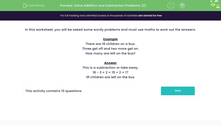# Solve A Mixture of Addition and Subtraction Word Problems

In this worksheet, students will answer word problems using addition and subtraction.Key stage:  KS 1

Curriculum topic:   Number: Addition and Subtraction

Curriculum subtopic:   Add and Subtract to 20

Difficulty level:#### Worksheet Overview

In this activity, you will be asked some wordy problems and must use maths to work out the answers.

Example

There are 18 children on a bus.

3 get off and 2 more get on.

How many are left on the bus?

This uses both subtraction and addition!

18 - 3 + 2 = 15 + 2 = 17

17 children are left on the busDon't be put off by all the words, there will be some sort of calculation to do in there somewhere!

Learning how to read a question and work out what sort of calculation is needed is a really important skill. So, if you can get to grips with these, you will be a super maths wizard!Are you ready to have a go?

### What is EdPlace?

We're your National Curriculum aligned online education content provider helping each child succeed in English, maths and science from year 1 to GCSE. With an EdPlace account you’ll be able to track and measure progress, helping each child achieve their best. We build confidence and attainment by personalising each child’s learning at a level that suits them.

Get started